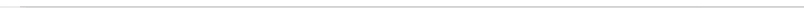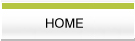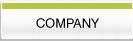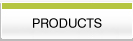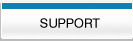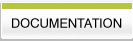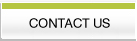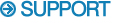Old Format Reading and writing Old Format data files. Felix old format data files were designed to have a very simple format with an optional header if desired. In its simplest form an old format data file would consist of a four byte integer specifying the number of complex points in the file followed by a series of four byte floating point values which make up the data. This simple format is often used by customers when a conversion program is required to convert from or to Felix format from some external format. One complication however is that the Felix old format is typically read or written by FORTRAN routines and will contain some additional byte offset values that are present due to the fact that the file was written out by FORTRAN. The following information should be taken into account by users wishing to read or write old format files with programs other than FORTRAN. A typical old format data file with parameters might be written out by FORTRAN like this: The following example assumes a 1024 complex data point file (2048 total data point values) with a 32 point parameter array (np=16=32/2). word type typ_value description 1 integer(4 byte) -16 np-number of parameters/2 2 integer(4 byte) 1024 first parameter complex points(total_points/2) 3 integer(4 byte) 1 second parameter 0=real,1=complex 4 integer(4 byte) 0 third parameter 0=fid,1=spectrum . . . 33 last parameter slot 34 integer(4 byte) 1024 number of complex points(total_points/2) 35 real(4 byte) xxxx first data point-real component 36 real(4 byte) xxxx first data point-imaginary component . . . 2081 real(4 byte) xxxx last data point-real component 2082 real(4 byte) xxxx last data point-imaginary component But because the file is written by FORTRAN the above representation will not be exactly what is written to the file. FORTRAN will write out a block offset value at the beginning and end of each write operation. The above information would generally be written out in two write statements. The first statement would write the number_of_parameters/2 value and the parameter array. The second write statement would write the total_points/2 parameter and the array of data values. Thus the file as written out by FORTRAN would contain 4 extra integer values(4 bytes each) which represent the block offsets. Thus the actual data on the disk might look something like this: word type typ_value description 1 integer(4 byte) 132 number of bytes in parameter block(32*4+4) 2 integer(4 byte) -16 np-number of parameters/2 3 integer(4 byte) 1024 first parameter complex points(total_points/2) 4 integer(4 byte) 1 first parameter 0=real,1=complex 5 integer(4 byte) 0 second parameter 0=fid,1=spectrum . . . 34 last parameter slot 35 integer(4 byte) 132 number of bytes in parameter block(32*4+4) 36 integer(4 byte) 8196 number of bytes in data block(2048*4+4) 37 integer(4 byte) 1024 number of complex points(total_points/2) 38 real(4 byte) xxxx first data point-real component 39 real(4 byte) xxxx first data point-imaginary component . . . 2084 real(4 byte) xxxx last data point-real component 2085 real(4 byte) xxxx last data point-imaginary component 2086 integer(4 byte) 8196 number of bytes in data block(2048*4+4) Note that the parameter block of data (the value for the number of parameters divided by 2 and the parameter array itself) is now bracketed by a pair of block offset values written by FORTRAN. The value for these block offset values is 132 in this case. This is the number of bytes in the 33 words that make up this parameter part of the data. Note that the data section of the file is also bracketed by two values (8196) which represent the number of bytes in this part of the file. Old format data files often have a parameter block consisting of 256 parameter values. Note that the number in the file which represents the number of parameters has a value which is the number of parameters divided by 2. Thus the file would have an np parameter of 128. This number is then negated for files which contain parameters. The actual data file would have an np parameter of -128. The following is a section out of the "od -S" output from a old format data file with 1024 complex data points (2048 total points). 0000000 0000001028 -0000000128 0000001024 0000000001 0000020 0000000000 0000000001 0000000000 0000000000 0000040 0000000000 0000000000 0000000000 0000000000 * 0000100 0000000000 0000000000 1166610350 1140461797 0000120 0000000000 0000000000 0000000000 0000000000 * 0000600 0000008192 0000000001 0000000000 0000000001 0000620 0000000000 0000000000 0000000000 0000000000 * 0000700 1166610350 1140461797 0000000000 0000000000 0000720 0000000000 0000000000 0000000000 0000000000 * 0002000 0000000000 0000000000 0000001028 0000008196 0002020 0000001024 -0913509496 1223514880 -0914476304 0002040 1222474400 -0971747328 -0951802880 -0916812288 0002060 1226275200 1210682048 1233603344 1226471728 * 0021720 1165799424 -1003913216 1160810496 -1001144320 0021740 1175302144 -0986939392 1159045120 -1025245184 0021760 1156956160 -0999604224 1175241728 -0984010752 0022000 1150951424 -0995205120 1162887168 -0993099776 0022020 1172465664 0000008196 0022030 To interpret an old format data file you could read and ignore the first 4 byte integer. Then read the next 4 byte integer. If this value is negative it means that the following values are parameters. Multiply this number by 2 (and take the absolute value) to get the number of parameter values which follow. Read in these parameter values. Then ignore the next two 4 byte integers because these will be byte offset values. Then read the next 4 byte value as an integer. This value represents the number of complex points in the data. Multiply this value by 2 and read in this many floating point data values. This is a general overview of how to read an old format data file. You should also take a look at a file called oldformat.doc which describes some of this in more detail and gives some code fragments and examples of how the old format data files are read and written. This file is located in the \$BIOSYM/gifts/felix/newformat directory if you installed the gift files or you can also find this file in the corresponding directory on your gifts and tutorials CD. •   Back to FAQ's    •   Contact us www.FelixNMR.com © 2007